# Texas Go Math Grade 1 Lesson 8.1 Answer Key Choose an Operation

Refer to our Texas Go Math Grade 1 Answer Key Pdf to score good marks in the exams. Test yourself by practicing the problems from Texas Go Math Grade 1 Lesson 8.1 Answer Key Choose an Operation.

## Texas Go Math Grade 1 Lesson 8.1 Answer Key Choose an Operation

Essential Question
How con you choose when to add and when to subtract to solve a problem?

Listen to the problem. Useor objects to solve. Draw a picture to show your work.
___ math games

For The Teacher

• Read the following problem. Kira has 16 computer games. There are 8 adventure games. The rest are math games. How many math games does Kira have?

Explanation:
16 – 8 = 8
Kira has 8 math games.Math Talk

Mathematical Processes
How did you solve this problem? Explain your strategy.
Explanation:
Kira has 16 computer games. There are 8 adventure games. The rest are math games.
16 – 8 = 8
8 math games that Kira have

Model and Draw
Mary sees 8 squirrels. Jack sees 9 more squirrels than Mary. How many squirrels does Jack see? Do you add or subtract to solve?

Explain how you chose a strategy to solve the problem.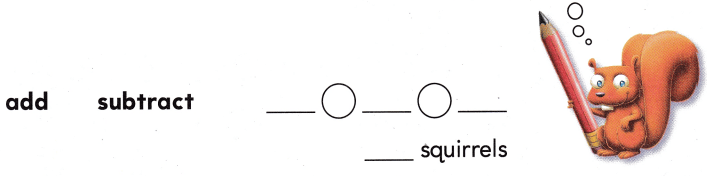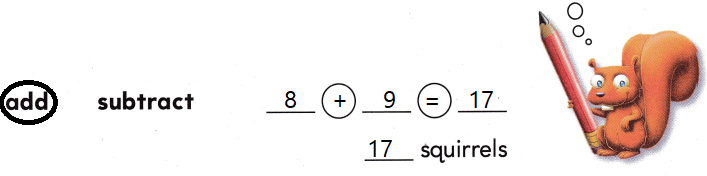Explanation:
8 + 9 = 17 squirrels that jack see

Share and Show

Write a number sentence to solve.

Question 1.
Hanna has 5 markers. Owen has 9 more markers than Hanna. How many markers does Owen have?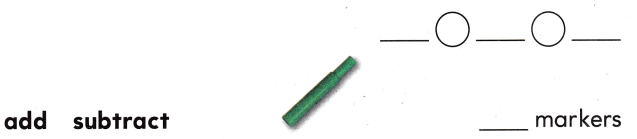Explanation:
Hanna has 5 markers. Owen has 9 more markers than Hanna.
5 + 9 = 14
Owen has 14 markers now.

Question 2.
Angel has 13 apples. He gives some away. Then there were 5 apples. How many apples does he give away?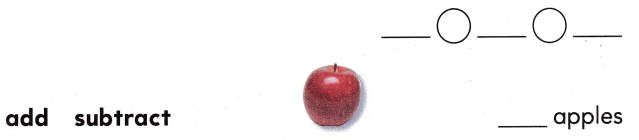Explanation:
Angel has 13 apples. He gives some away. Then there were 5 apples.
13 – 5 = 8
he gave away 8 apples.

Question 3.
Deon has 18 blocks. He builds a house with 9 of the blocks. How many blocks does Deon have now?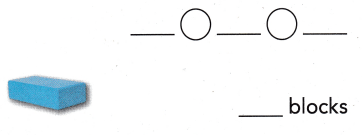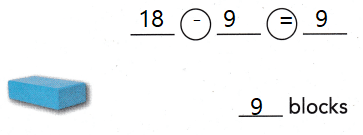Explanation:
Deon has 18 blocks. He builds a house with 9 of the blocks.
18 – 9 = 9
Deon has 9 blocks now.

Problem Solving

Write a number sentence to solve.

Question 4.
Rob sees 5 raccoons. Talia sees 4 more raccoons than Rob. How many raccoons do they see?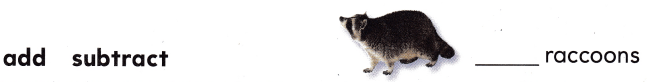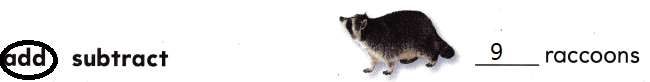Explanation:
Rob sees 5 raccoons. Talia sees 4 more raccoons than Rob.
5 + 4 = 9
They see 9 raccoons.

Write or draw to solve.

Question 5.
James has 4 big markers and 7 small markers. How many markers does he have?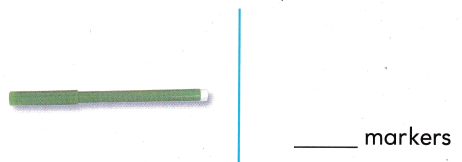Explanation:
James has 4 big markers and 7 small markers.
4 + 7 = 11
they have 11 markers in all

Question 6.
H.O.T. Sam has 9 baseball cards. She gets one card from Mom. She wants to have 17 cards. How many more cards does she need?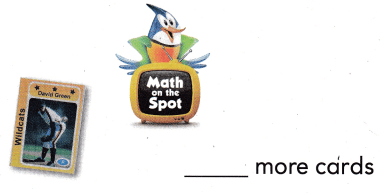Explanation:
Sam has 9 baseball cards. She gets one card from Mom.
9 + 1 = 10
She wants to have 17 cards.
17 – 10 = 7
she need 7 more cards

Question 7.
H.O.T. Multi-Step Annie gets 15 pennies on Monday. She gets 1 more each day. How many pennies does she have on Friday?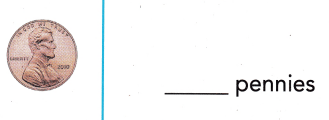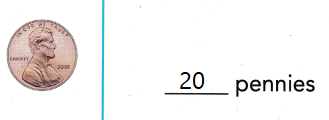Explanation:
15 + 5 = 20
Monday to Friday there are 5 days
she has 20 on Friday

Question 8.
Use Tools A tree has 9 trunks. 5 trunks are large. The rest are small. Which number sentence shows how many trunks are small?
(A) 5 – 4 = 1
(B) 9 – 5 = 4
(C) 9 + 5 = 14
Explanation:
A tree has 9 trunks. 5 trunks are large.
9 – 5 = 4
4 trunks are small.

Question 9.
Morgan bought 8 lemons. She gave 3 lemons to her friends. Which number sentence shows how many lemons Morgan has now?(A) 8 + 3 = 11
(B) 3 – 3 = 0
(C) 8 – 3 = 5
Explanation:
Morgan bought 8 lemons. She gave 3 lemons to her friends.
8 – 3 = 5
Morgan has 5 lemons now.

Question 10.
Multi-Step Jared has 7 toy trains. He gets 4 more from his mother. Then he gives 3 toy trains to his brother. How many toy trains does Jared have now?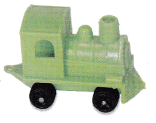(A) 8
(B) 11
(C) 4
Explanation:
Jared has 7 toy trains. He gets 4 more from his mother.
7 + 4 = 11
Then he gives 3 toy trains to his brother.
11 – 3 = 8
Jared has 8 toys now

Question 11.
Texas Test Prep There are 15 kittens. 6 are black. The rest are white. Which shows how many white kittens there are?
(A) 15 – 6 = 9
(B) 10 + 5 = 15
(C) 9 – 6 = 3
Explanation:
There are 15 kittens. 6 are black. The rest are white.
15 – 6 = 9
so, 9 cats are white

Take Home Activity

• Ask your child o write a number sentence that could be used to solve the following problem: Eli has a box with 12 eggs. His other box has no eggs. How many eggs are in both boxes?
Explanation:
12 + 0 = 12
In both the boxes combined had 12 eggs.

### Texas Go Math Grade 1 Lesson 8.1 Homework and Practice Answer Key

Circle add or subtract. Write a number sentence to solve.

Question 1.
Kara has 17 books. Sam has 8 fewer books than Kara. How many books does Sam have?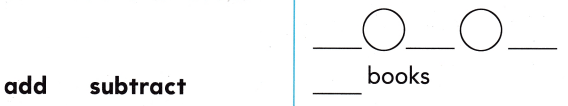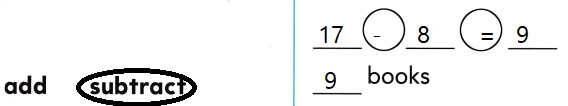Explanation:
Kara has 17 books. Sam has 8 fewer books than Kara.
17 – 8 = 9
Sam has 9 books now

Question 2.Explanation:
6 + 3 = 9
9 + 6 = 15

Problem Solving

Write or draw to solve.

Question 3.
Multi-Step Isabella needs 16 muffins. She makes 9 banana muffins. Lucas gives her 2 more. How many more muffins does she need? Explain the strategy you used to solve the problem.
___ more muffins
Explanation:
9 + 2 = 11
16 – 11= 5

Texas Test Prep

Lesson Check

Question 4.
Zoe has 6 toy cars. Gabe has 9 more toy cars than Zoe. Which number sentence matches the picture?(A) 6 + 3 = 9
(B) 9 – 6 = 3
(C) 6 + 9 = 15
Explain the strategy you used to solve the problem.
Explanation:
Zoe has 6 toy cars. Gabe has 9 more toy cars than Zoe.
6 + 9 = 15 matches the picture.

Question 5.
There are 13 animals. 5 are dogs. The rest are cats. Which number sentence matches the picture?Explain the strategy you used to solve the problem.
Answer: 13 – 5 = 8
Explanation:
13 – 5 = 8
There are 8 cats

Question 6.
Multi-Step Katie has 5 small balls. She buys 9 more. Then she gives 6 to her friend. How many small balls does Katie have now?
(A) 8
(B) 14
(C) 9